Open in App
Not now

# Introduction to Amortized Analysis

• Difficulty Level : Medium
• Last Updated : 05 Jan, 2023

Amortized Analysis is used for algorithms where an occasional operation is very slow, but most of the other operations are faster. In Amortized Analysis, we analyze a sequence of operations and guarantee a worst-case average time that is lower than the worst-case time of a particularly expensive operation.
The example data structures whose operations are analyzed using Amortized Analysis are Hash Tables, Disjoint Sets, and Splay Trees.

Let us consider an example of simple hash table insertions. How do we decide on table size? There is a trade-off between space and time, if we make hash-table size big, search time becomes low, but the space required becomes high.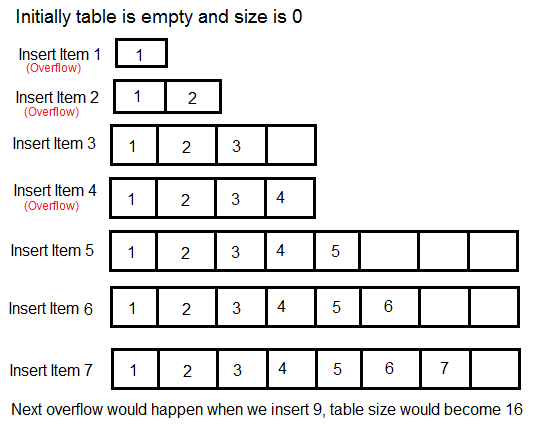The solution to this trade-off problem is to use Dynamic Table (or Arrays). The idea is to increase the size of the table whenever it becomes full. Following are the steps to follow when the table becomes full.
1) Allocate memory for larger table size, typically twice the old table.
2) Copy the contents of the old table to a new table.
3) Free the old table.

If the table has space available, we simply insert a new item in the available space.

What is the time complexity of n insertions using the above scheme?
If we use simple analysis, the worst-case cost of insertion is O(n). Therefore, the worst-case cost of n inserts is n * O(n) which is O(n2). This analysis gives an upper bound, but not a tight upper bound for n insertions as all insertions don’t take Θ(n) time.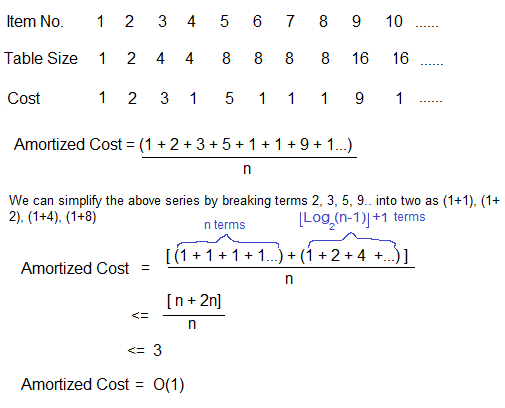So using Amortized Analysis, we could prove that the Dynamic Table scheme has O(1) insertion time which is a great result used in hashing. Also, the concept of the dynamic table is used in vectors in C++ and ArrayList in Java

Following are a few important notes.
1) Amortized cost of a sequence of operations can be seen as expenses of a salaried person. The average monthly expense of the person is less than or equal to the salary, but the person can spend more money in a particular month by buying a car or something. In other months, he or she saves money for the expensive month.

2) The above Amortized Analysis was done for Dynamic Array example is called Aggregate Method. There are two more powerful ways to do Amortized analysis called Accounting Method and Potential Method. We will be discussing the other two methods in separate posts.

3) The amortized analysis doesn’t involve probability. There is also another different notion of average-case running time where algorithms use randomization to make them faster and the expected running time is faster than the worst-case running time. These algorithms are analyzed using Randomized Analysis. Examples of these algorithms are Randomized Quick Sort, Quick Select and Hashing. We will soon be covering Randomized analysis in a different post.

Amortized analysis of insertion in Red-Black Tree

Let us discuss the Amortized Analysis of Red-Black Tree operations (Insertion) using the Potential Method.

To perform the amortized analysis of the Red-Black Tree Insertion operation, we use the Potential(or Physicist’s) method. For the potential method, we define a potential functionthat maps a data structure to a non-negative real value. An operation can result in a change of this potential.

Let us define the potential functionin the following manner:

(1)where n is a node of Red-Black Tree

Potential function,over all nodes of the red black tree.

Further, we define the amortized time of an operation as:

Amortized time= c +(h)(h)=(h’) –(h)
where h and h’ are the states of the Red-Black Tree before and after the operation respectively
c is the actual cost of the operation

The change in potential should be positive for low-cost operations and negative for high-cost operations.

A new node is inserted on a leaf of a red-black tree. We have the leaves of a red-black tree of any one of the following types: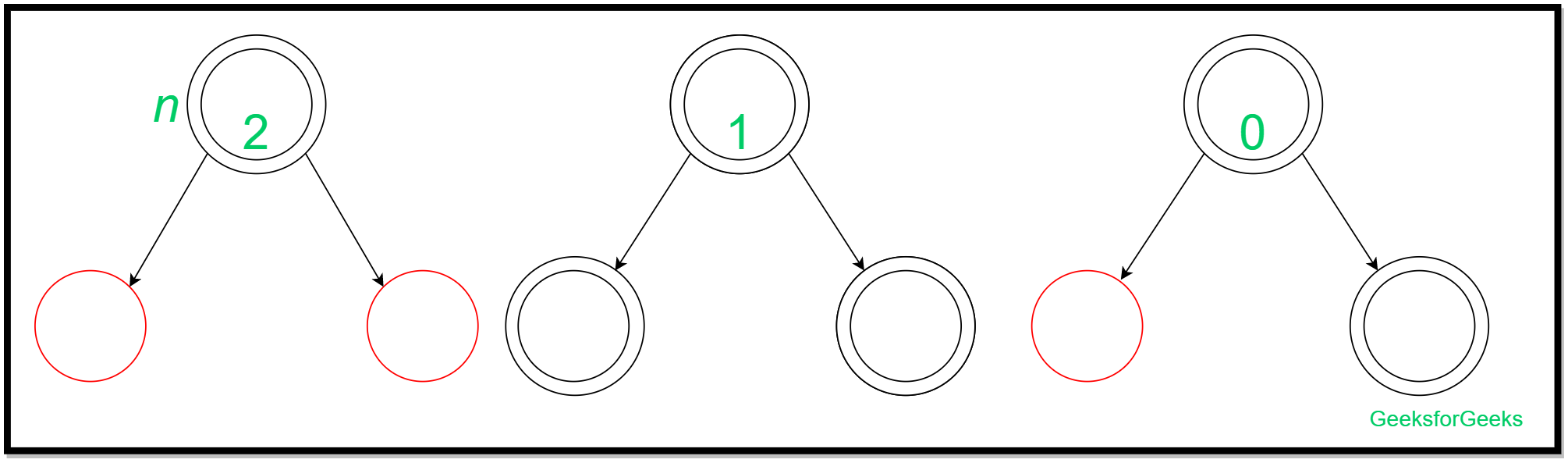The insertions and their amortized analysis can be represented as:
(1)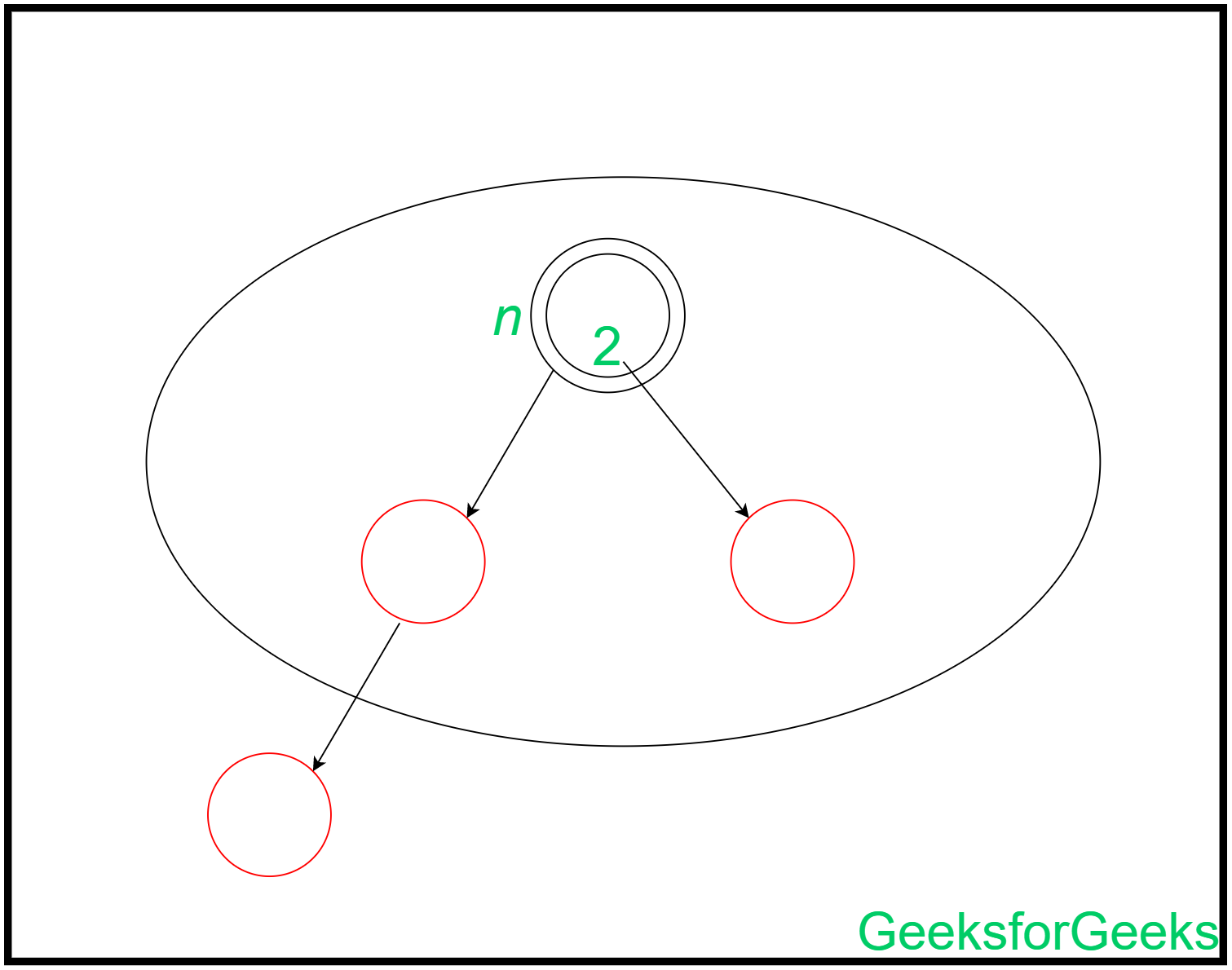This insertion is performed by first recoloring the parent and the other sibling(red). Then the grandparent and uncle of that leaf node are considered for further recoloring which leads to the amortized cost to be -1(when the grandparent of the leaf node is red), -2 (when uncle of the leaf is black and the grandparent is black) or +1 (when uncle of the leaf is red and grandparent is black). The insertion can be shown as: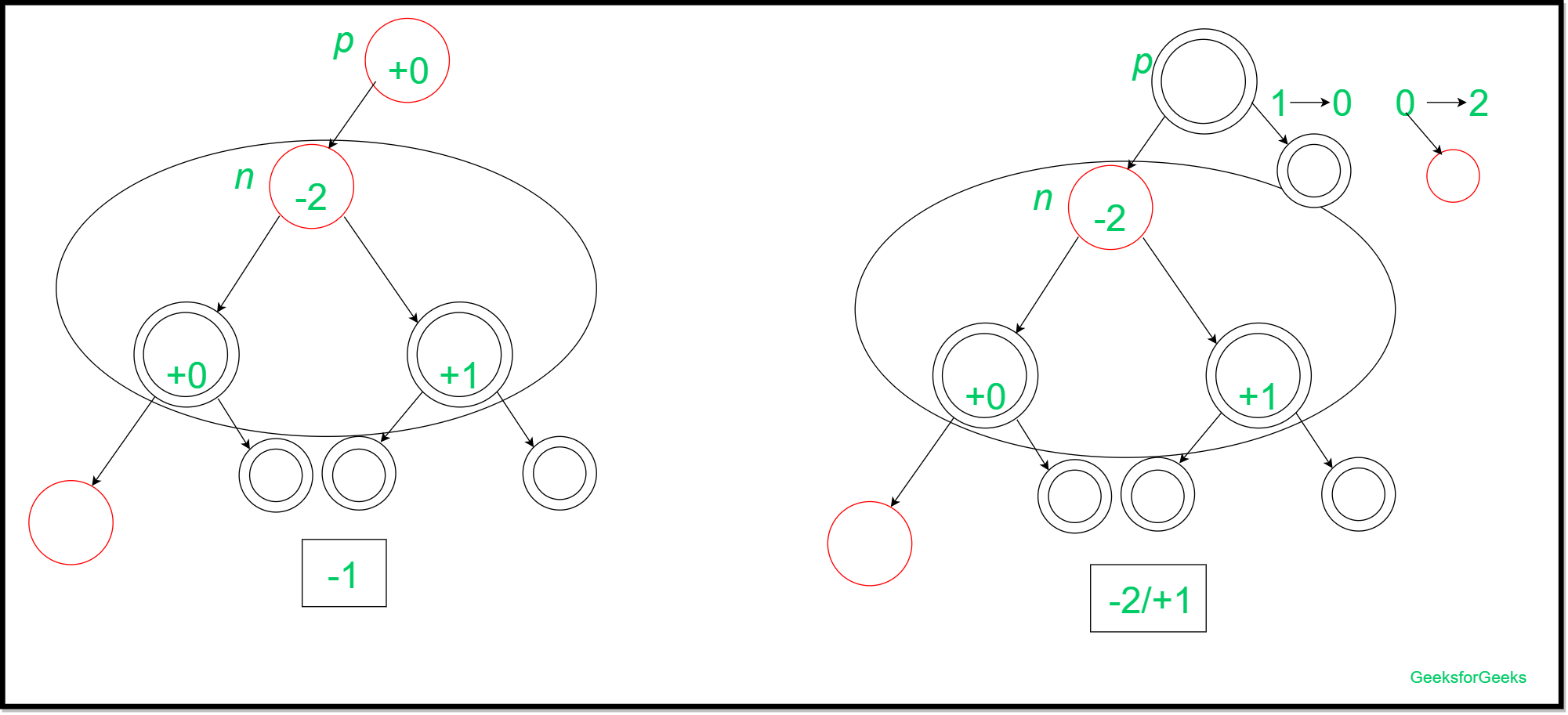(2)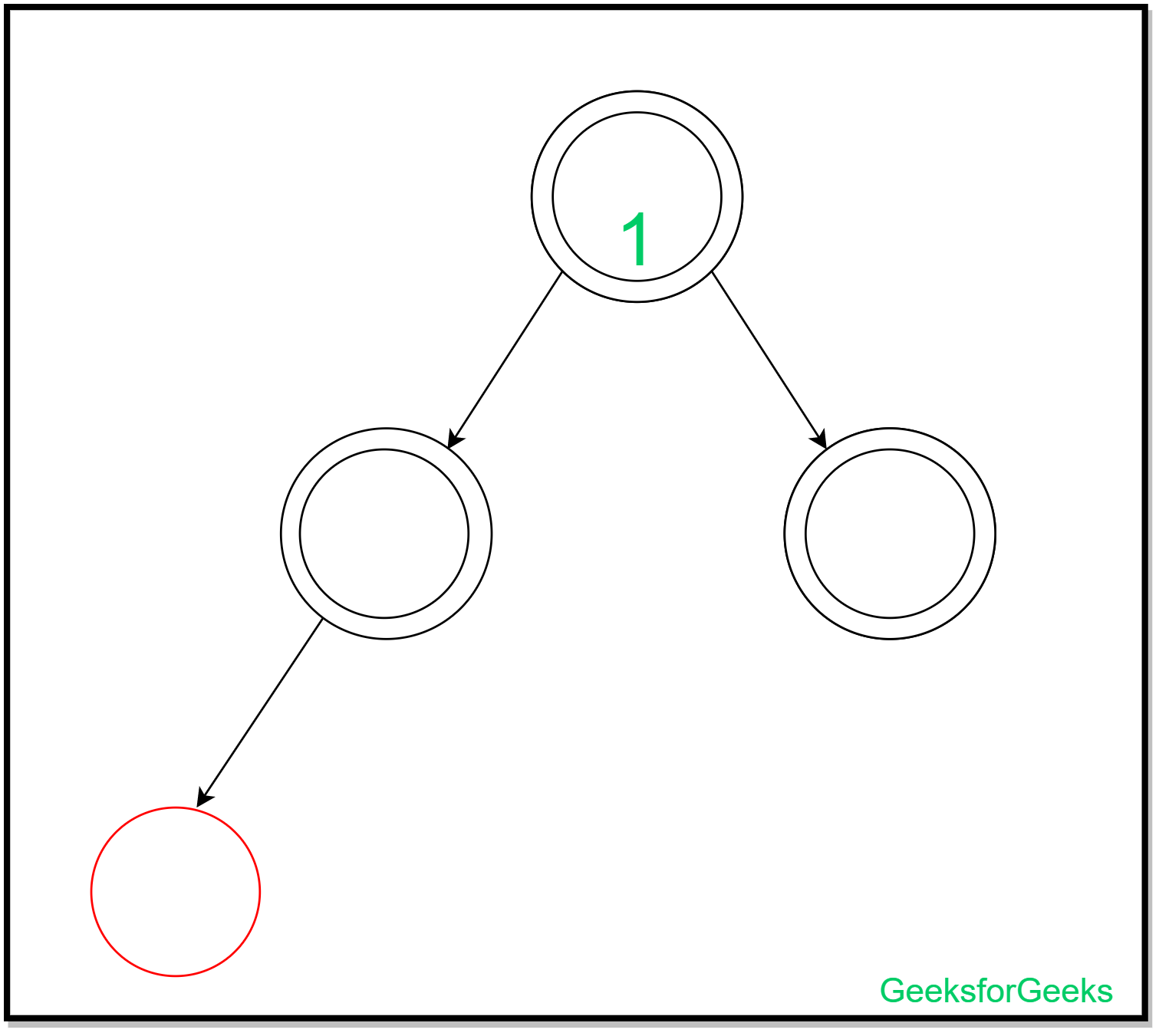In this insertion, the node is inserted without any changes as the black depth of the leaves remains the same. This is the case when the leaf may have a black sibling or does not have any sibling (since we consider the color of the color of null node to be black).

So, the amortized cost of this insertion is 0

(3)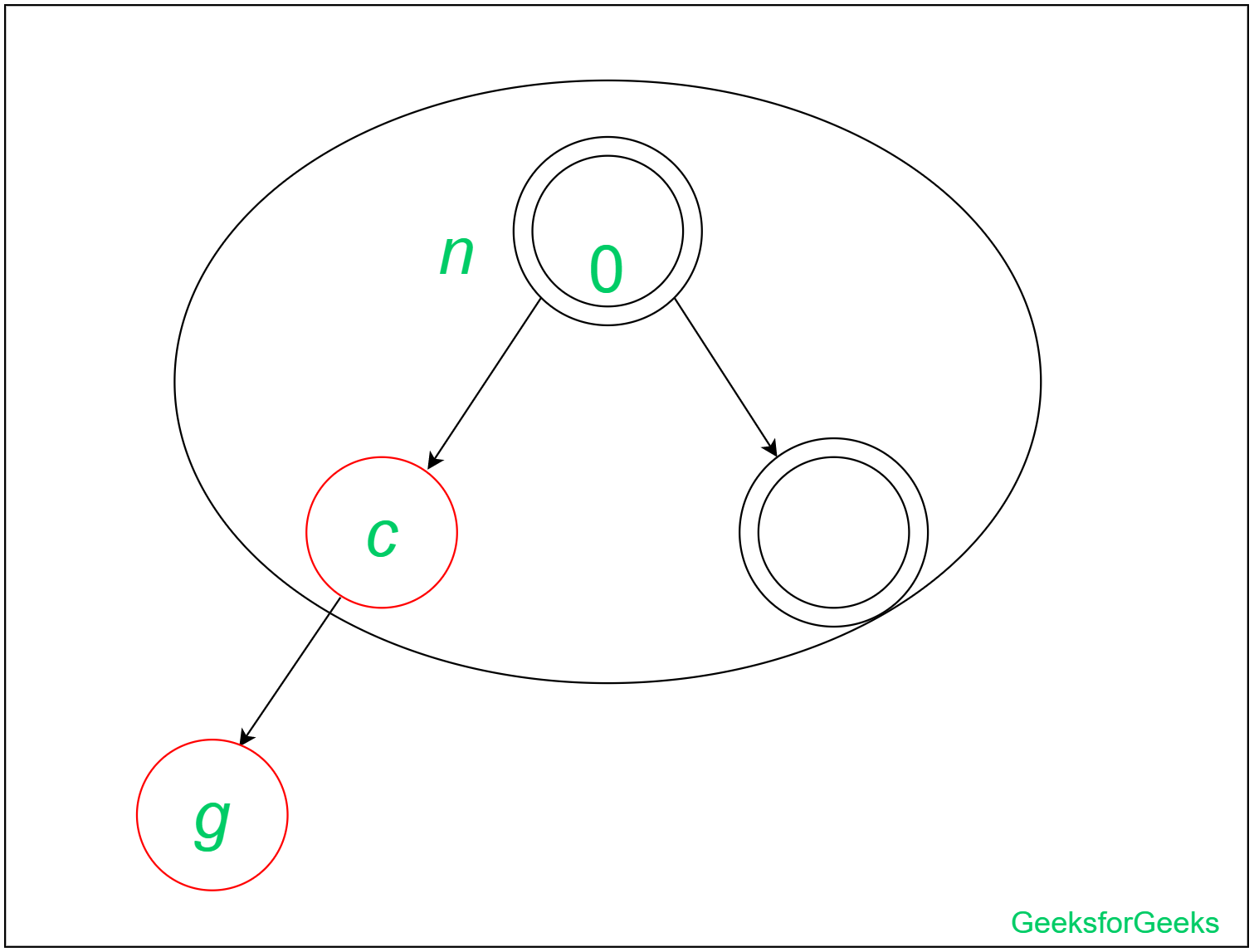In this insertion, we cannot recolor the leaf node, its parent, and the sibling such that the black depth stays the same as before. So, we need to perform a Left- Left rotation.

After rotation, there are no changes when the grandparent of g(the inserted node) is black. Also, for the case of the Red Grandparent of g(the inserted node), we do not have any changes. So, the insertion is completed with amortized cost= +2. The insertion has been depicted below: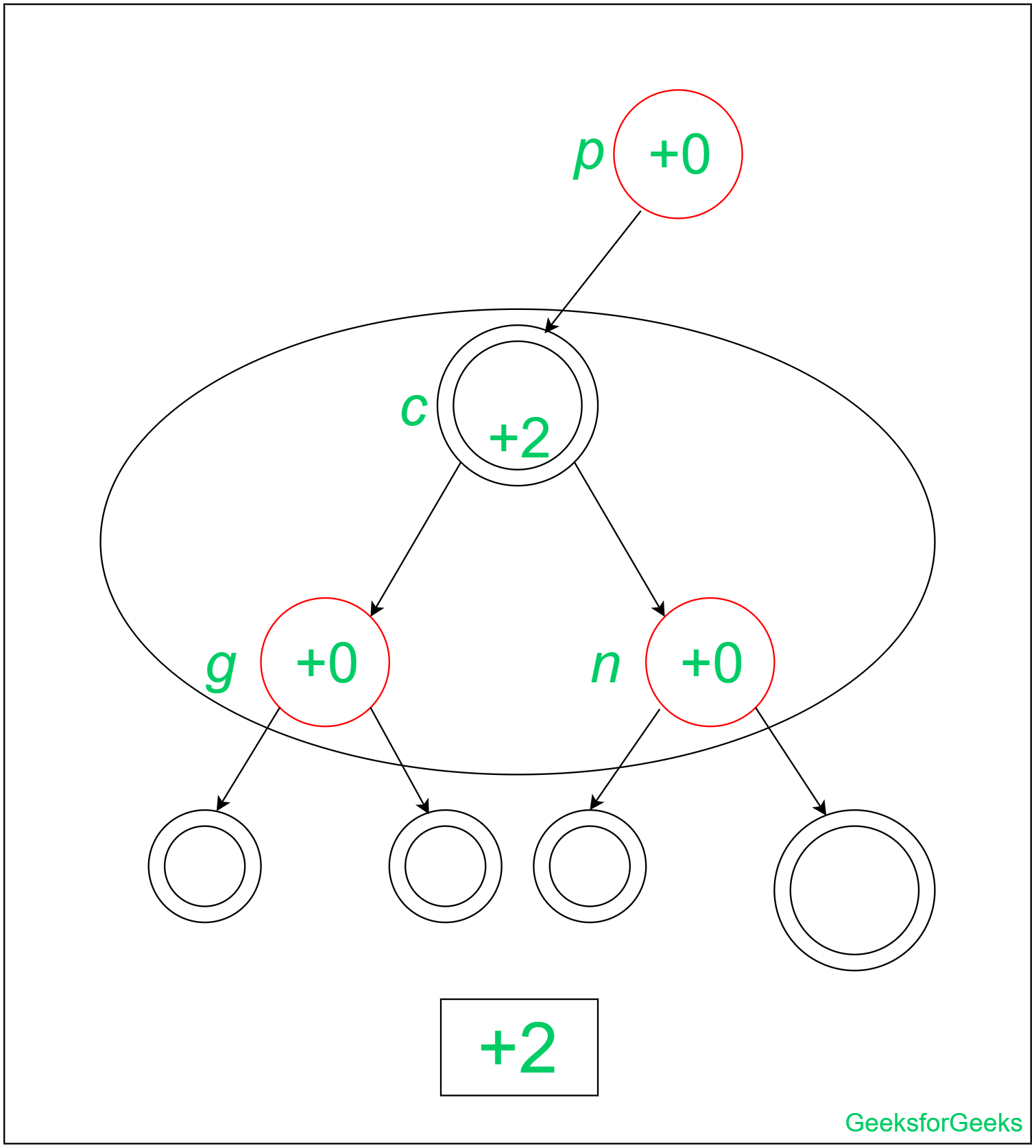After calculating these particular amortized costs at the leaf site of a red-black tree we can discuss the nature of the total amortized cost of insertion in a red-black tree. Since this may happen that two red nodes may have a parent-child relationship till the root of the red-black tree.

So in the extreme(or corner) case, we reduce the number of black nodes with two red children by 1, and we at most increase the number of black nodes with no red children by 1, leaving a net loss of at most 1 to the potential function. Since one unit of potential pays for each operation therefore(h)where n is the total number of nodes

Thus, the total amortized cost of insertion in the Red-Black Tree is O(n)

For any doubts regarding insertions in a red-black tree, you may refer to Insertions in Red-Black Tree

For more details, please refer: Design and Analysis of Algorithms.